# RD Sharma Solutions for Class 10 Maths Chapter 3 Pair of Linear Equations in Two Variables Exercise 3.6

The linear pair of equations in two variables has got many applications in science and statistics. This is to say the applications to word problems are enormous and extensively used in everyday life. The RD Sharma Solutions Class 10 are vital tools for students to acquire strong conceptual knowledge and boost their confidence in taking tests. Students can refer to the RD Sharma Solutions for Class 10 Maths Chapter 3 Pair of Linear Equations in Two Variables Exercise 3.6 PDF given below for any help regarding this exercise.

## RD Sharma Solutions for Class 10 Maths Chapter 3 Pair of Linear Equations in Two Variables Exercise 3.6 Download the PDF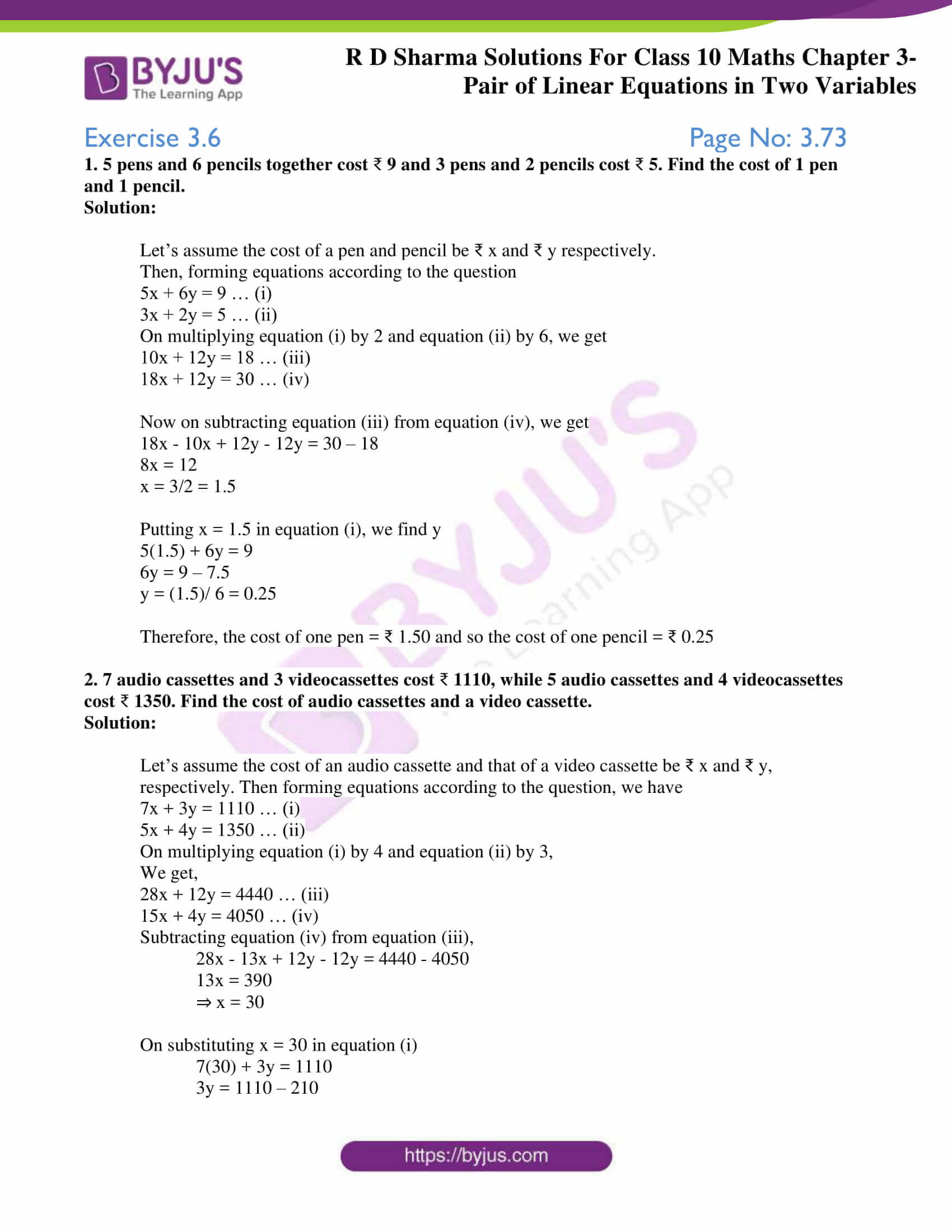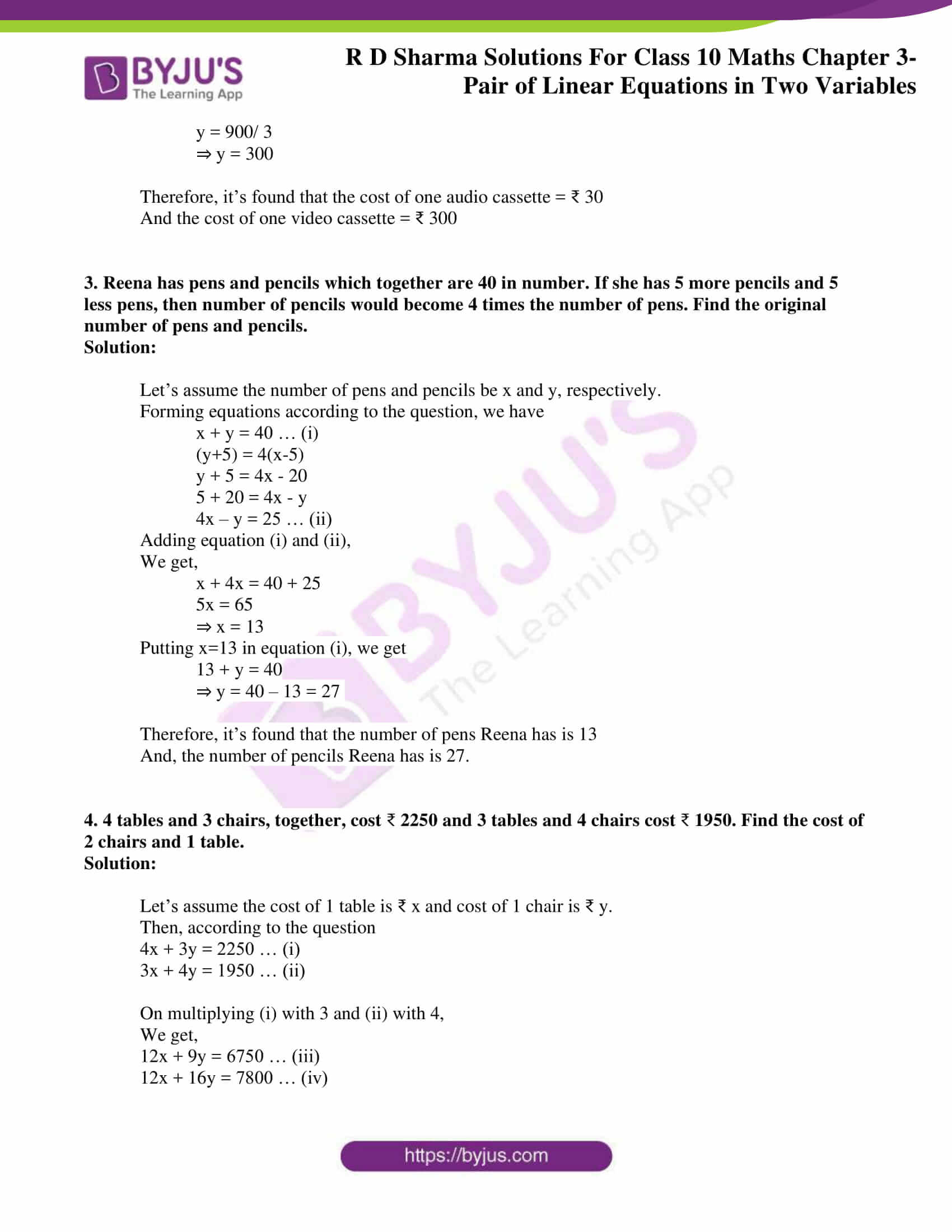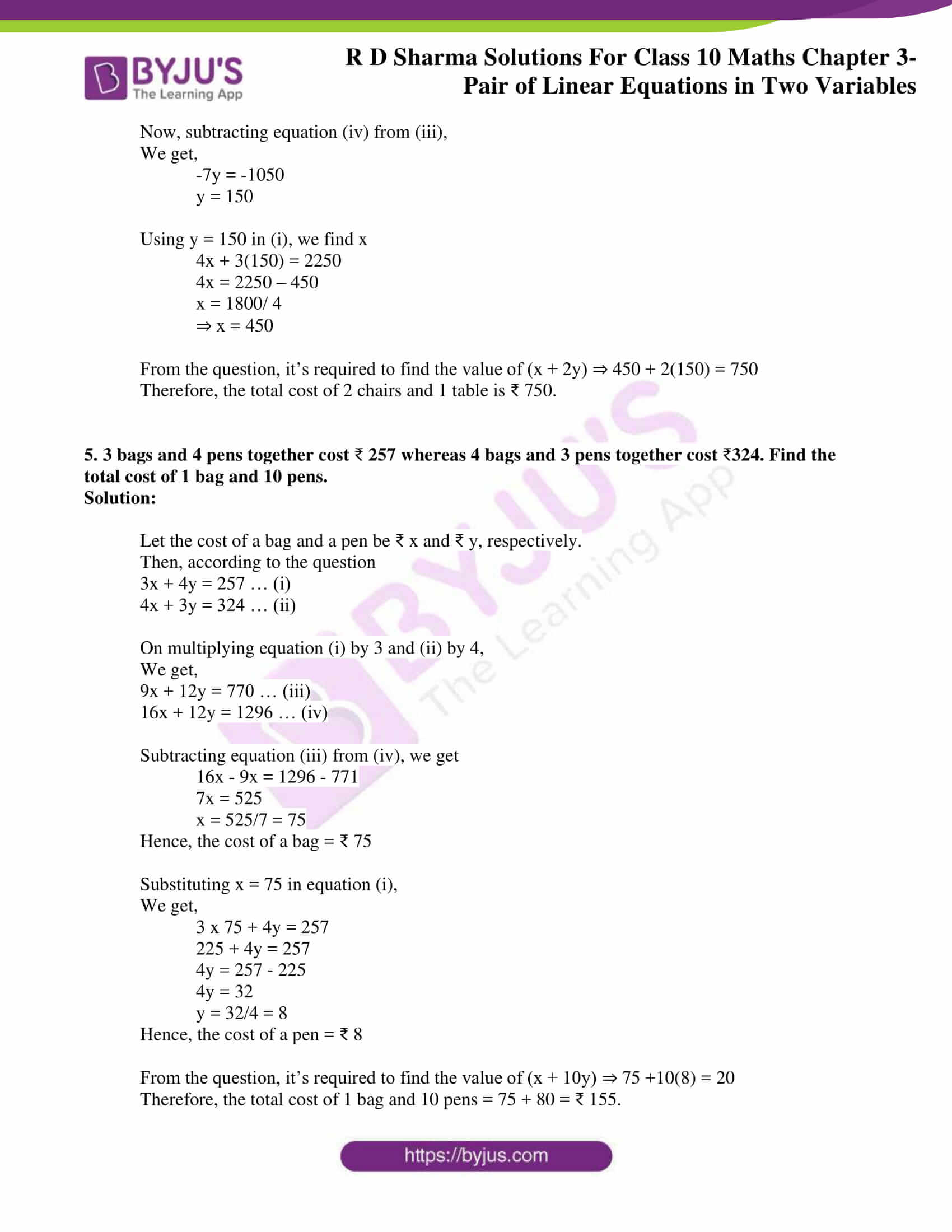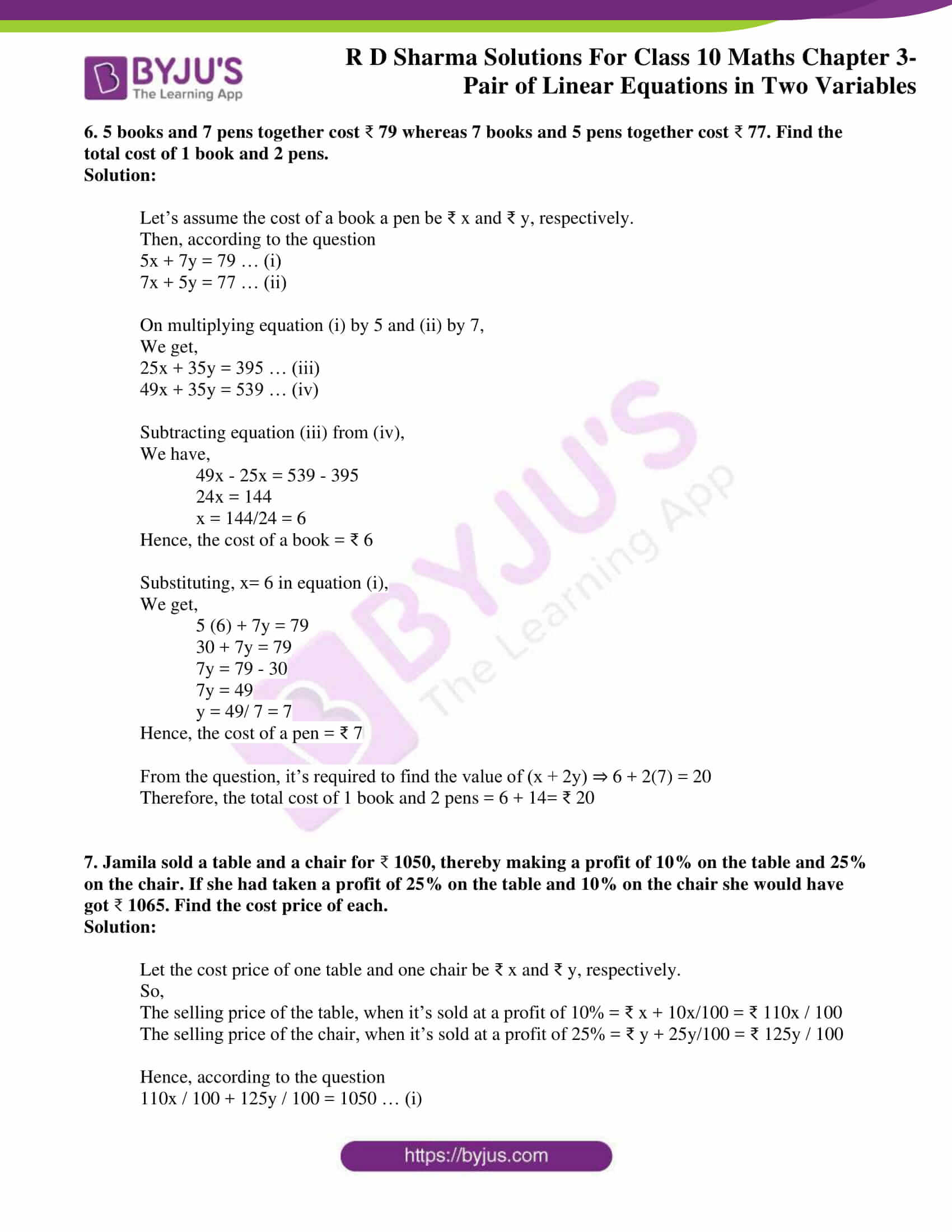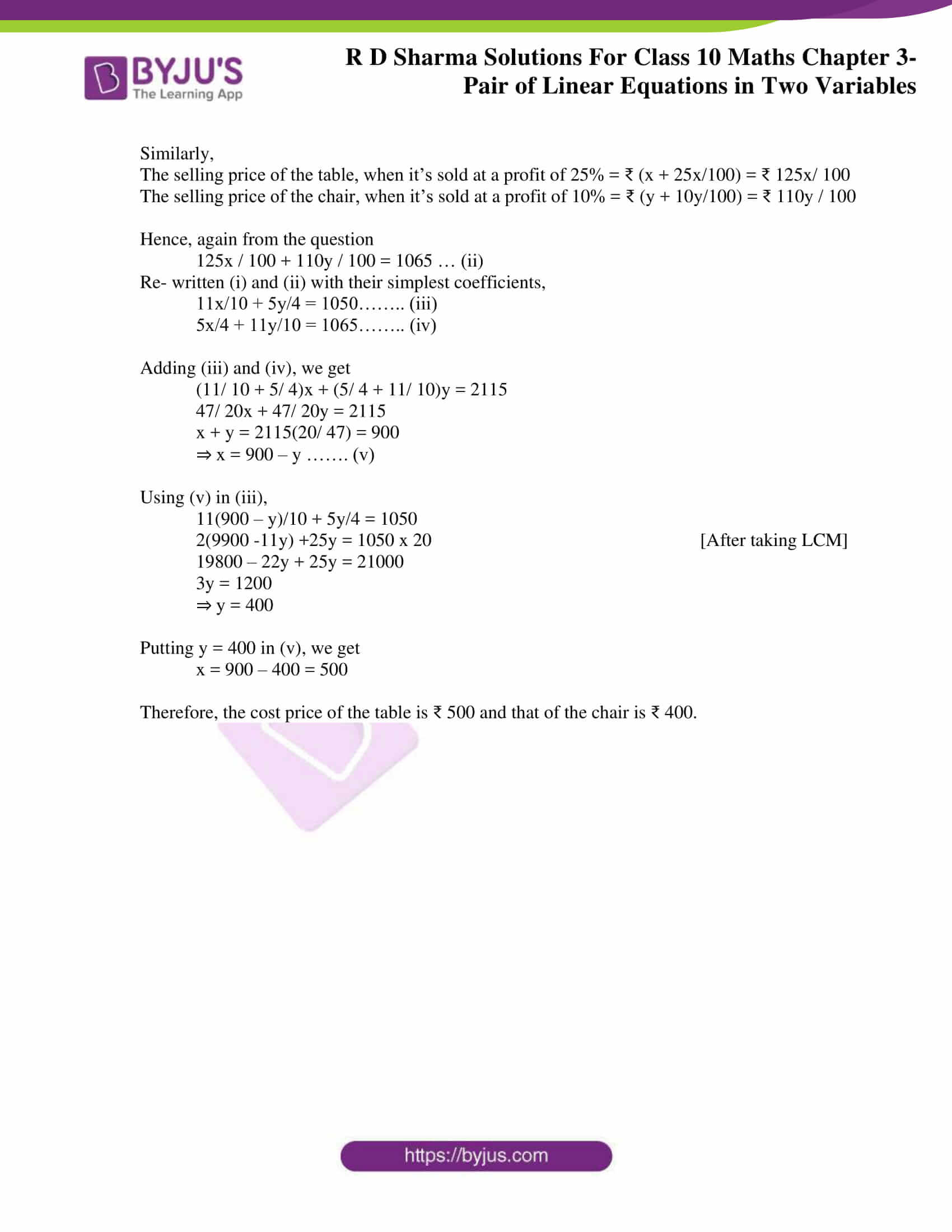### Access RD Sharma Solutions for Class 10 Maths Chapter 3 Pair of Linear Equations in Two Variables Exercise 3.6

1. 5 pens and 6 pencils together cost 9, and 3 pens and 2 pencils cost 5. Find the cost of 1 pen and 1 pencil.

Solution:

Let’s assume the cost of a pen and pencil is ₹ x and ₹ y, respectively.

Then, forming equations according to the question,

5x + 6y = 9 … (i)

3x + 2y = 5 … (ii)

On multiplying equation (i) by 2 and equation (ii) by 6, we get

10x + 12y = 18 … (iii)

18x + 12y = 30 … (iv)

Now by subtracting equation (iii) from equation (iv), we get

18x – 10x + 12y – 12y = 30 – 18

8x = 12

x = 3/2 = 1.5

Putting x = 1.5 in equation (i), we find y.

5(1.5) + 6y = 9

6y = 9 – 7.5

y = (1.5)/ 6 = 0.25

Therefore, the cost of one pen = ₹ 1.50, and so the cost of one pencil = ₹ 0.25

2. 7 audio cassettes and 3 videocassettes cost 1110, while 5 audio cassettes and 4 videocassettes cost 1350. Find the cost of audio cassettes and a video cassette.

Solution:

Let’s assume the cost of an audio cassette, and that of a video cassette be ₹ x and ₹ y, respectively. Then, forming equations according to the question, we have

7x + 3y = 1110 … (i)

5x + 4y = 1350 … (ii)

On multiplying equation (i) by 4 and equation (ii) by 3,

We get

28x + 12y = 4440 … (iii)

15x + 4y = 4050 … (iv)

Subtracting equation (iv) from equation (iii),

28x – 13x + 12y – 12y = 4440 – 4050

13x = 390

⇒ x = 30

On substituting x = 30 in equation (I),

7(30) + 3y = 1110

3y = 1110 – 210

y = 900/ 3

⇒ y = 300

Therefore, it’s found that the cost of one audio cassette = ₹ 30

And the cost of one video cassette = ₹ 300

3. Reena has pens and pencils, which together are 40 in number. If she has 5 more pencils and 5 less pens, then the number of pencils would become 4 times the number of pens. Find the original number of pens and pencils.

Solution:

Let’s assume the number of pens and pencils is x and y, respectively.

Forming equations according to the question, we have

x + y = 40 … (i)

(y+5) = 4(x-5)

y + 5 = 4x – 20

5 + 20 = 4x – y

4x – y = 25 … (ii)

We get

x + 4x = 40 + 25

5x = 65

⇒ x = 13

Putting x=13 in equation (i), we get

13 + y = 40

⇒ y = 40 – 13 = 27

Therefore, it’s found that the number of pens Reena has is 13.

And the number of pencils Reena has is 27.

4. 4 tables and 3 chairs, together, cost 2250, and 3 tables and 4 chairs cost 1950. Find the cost of 2 chairs and 1 table.

Solution:

Let’s assume the cost of 1 table is ₹ x, and the cost of 1 chair is ₹ y.

Then, according to the question,

4x + 3y = 2250 … (i)

3x + 4y = 1950 … (ii)

On multiplying (i) with 3 and (ii) with 4,

We get,

12x + 9y = 6750 … (iii)

12x + 16y = 7800 … (iv)

Now, subtracting equation (iv) from (iii),

We get,

-7y = -1050

y = 150

Using y = 150 in (i), we find x

4x + 3(150) = 2250

4x = 2250 – 450

x = 1800/ 4

⇒ x = 450

From the question, it’s required to find the value of (x + 2y) ⇒ 450 + 2(150) = 750

Therefore, the total cost of 2 chairs and 1 table is ₹ 750.

5. 3 bags and 4 pens together cost 257, whereas 4 bags and 3 pens together cost 324. Find the total cost of 1 bag and 10 pens.

Solution:

Let the cost of a bag and a pen be ₹ x and ₹ y, respectively.

Then, according to the question,

3x + 4y = 257 … (i)

4x + 3y = 324 … (ii)

On multiplying equation (i) by 3 and (ii) by 4,

We get,

9x + 12y = 770 … (iii)

16x + 12y = 1296 … (iv)

Subtracting equation (iii) from (iv), we get

16x – 9x = 1296 – 771

7x = 525

x = 525/7 = 75

Hence, the cost of a bag = ₹ 75

Substituting x = 75 in equation (i),

We get,

3 x 75 + 4y = 257

225 + 4y = 257

4y = 257 – 225

4y = 32

y = 32/4 = 8

Hence, the cost of a pen = ₹ 8

From the question, it’s required to find the value of (x + 10y) ⇒ 75 +10(8) = 20

Therefore, the total cost of 1 bag and 10 pens = 75 + 80 = ₹ 155

6. 5 books and 7 pens together cost 79, whereas 7 books and 5 pens together cost 77. Find the total cost of 1 book and 2 pens.

Solution:

Let’s assume the cost of a book and a pen be ₹ x and ₹ y, respectively.

Then, according to the question,

5x + 7y = 79 … (i)

7x + 5y = 77 … (ii)

On multiplying equation (i) by 5 and (ii) by 7,

We get,

25x + 35y = 395 … (iii)

49x + 35y = 539 … (iv)

Subtracting equation (iii) from (iv),

We have,

49x – 25x = 539 – 395

24x = 144

x = 144/24 = 6

Hence, the cost of a book = ₹ 6

Substituting x= 6 in equation (i),

We get

5 (6) + 7y = 79

30 + 7y = 79

7y = 79 – 30

7y = 49

y = 49/ 7 = 7

Hence, the cost of a pen = ₹ 7

From the question, it’s required to find the value of (x + 2y) ⇒ 6 + 2(7) = 20

Therefore, the total cost of 1 book and 2 pens = 6 + 14= ₹ 20

7. Jamila sold a table and a chair for 1050, thereby making a profit of 10% on the table and 25% on the chair. If she had taken a profit of 25% on the table and 10% on the chair, she would have got 1065. Find the cost price of each.

Solution:

Let the cost price of one table and one chair be ₹ x and ₹ y, respectively.

So,

The selling price of the table, when it’s sold at a profit of 10% = ₹ x + 10x/100 = ₹ 110x / 100

The selling price of the chair, when it’s sold at a profit of 25% = ₹ y + 25y/100 = ₹ 125y / 100

Hence, according to the question,

110x / 100 + 125y / 100 = 1050 … (i)

Similarly,

The selling price of the table, when it’s sold at a profit of 25% = ₹ (x + 25x/100) = ₹ 125x/ 100

The selling price of the chair, when it’s sold at a profit of 10% = ₹ (y + 10y/100) = ₹ 110y / 100

Hence, again from the question

125x / 100 + 110y / 100 = 1065 … (ii)

Re-written (i) and (ii) with their simplest coefficients,

11x/10 + 5y/4 = 1050…….. (iii)

5x/4 + 11y/10 = 1065…….. (iv)

Adding (iii) and (iv), we get

(11/ 10 + 5/ 4)x + (5/ 4 + 11/ 10)y = 2115

47/ 20x + 47/ 20y = 2115

x + y = 2115(20/ 47) = 900

⇒ x = 900 – y ……. (v)

Using (v) in (iii),

11(900 – y)/10 + 5y/4 = 1050

2(9900 -11y) +25y = 1050 x 20 [After taking LCM]

19800 – 22y + 25y = 21000

3y = 1200

⇒ y = 400

Putting y = 400 in (v), we get

x = 900 – 400 = 500

Therefore, the cost price of the table is ₹ 500, and that of the chair is ₹ 400.